# Matlab拟合工具箱的使用

1.打开 CFTOOL 工具箱。g"，点开"Curve Fitting Tool"，出现数据拟合工具界面，基本上所有的数据拟合和回归分析都可以在这里进行。也可以在命令窗口中直接输入”cftool”，打开工具箱。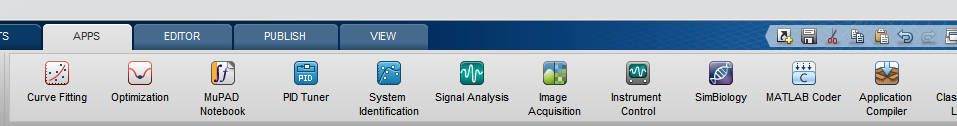2.输入两组向量 x，y。

x = [196,186, 137, 136, 122, 122, 71, 71, 70, 33];
y=[0.012605,0.013115,0.016866,0.014741,0.022353,0.019278,0.041803,0.038026,0.038128,0.088196];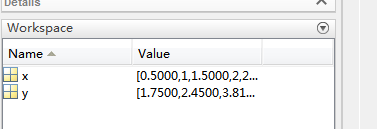3.数据的选取。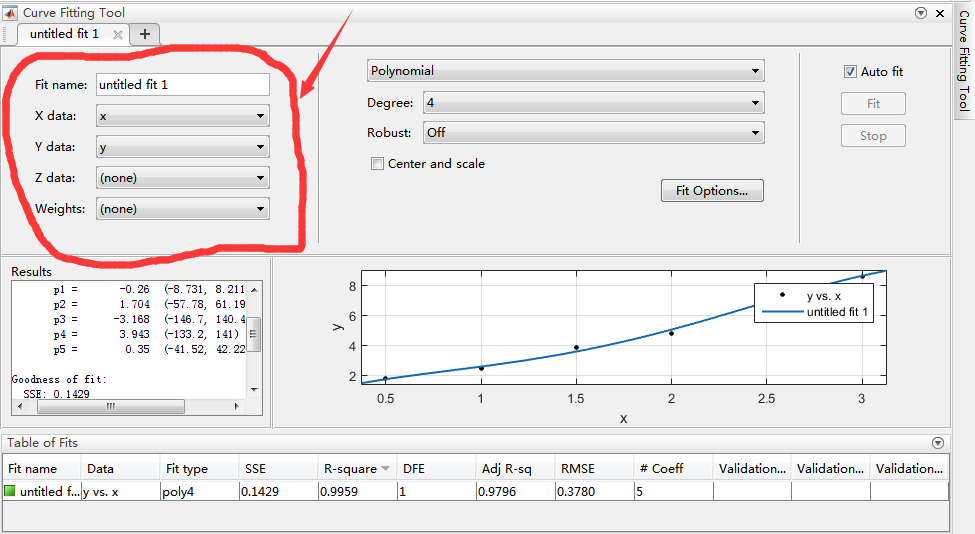4.曲线拟合(幂函数 power)。

Custom Equations 用户自定义函数
Expotential e指数函数
Fourier 傅立叶函数，含有三角函数
Gaussian 正态分布函数，高斯函数
Interpolant 插值函数，含有线性函数，移动平均等类型的拟合
Polynomial 多项式函数
Power 幂函数
Rational 有理函数
Smooth Spline （光滑插值或者光滑拟合，不太清楚）
Sum of sin functions 正弦函数类
Weibull 威布尔函数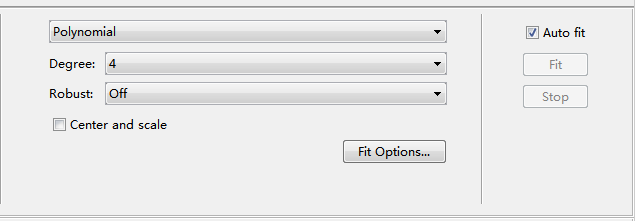5.拟合后的结果信息。

General model of sin1:
....... (函数形式）
Coefficients (with 95% conffidence range) (95%置信区间内的拟合常数）
a1=... ( ... ...) （等号后面是平均值，括号里是范围）
....
Godness of fit: (统计结果）
SSE: ... （方差）
R-squared: ... (决定系数，不知道做什么的）
RMSE: ... (标准差）

for example:

Linear model Poly4:
f(x) = p1*x^4 + p2*x^3 + p3*x^2 + p4*x + p5
Coefficients (with 95% confidence bounds):
p1 =       -0.26  (-8.731, 8.211)
p2 =       1.704  (-57.78, 61.19)
p3 =      -3.168  (-146.7, 140.4)
p4 =       3.943  (-133.2, 141)
p5 =        0.35  (-41.52, 42.22)

Goodness of fit:
SSE: 0.1429
R-square: 0.9959
RMSE: 0.378

7.图片导出。另外要说的是，如果想把这个拟合的图像导出的话，在 Curve
Fitting Tool窗口的 File菜单下选Print to Figure，此时弹出一个新的图像

p=polyfit(xdata,ydata,n) n 为选取的方法
a=polyval(p,xdata) 进行曲线拟合后计算所得到得值

plot(xdata,ydata,'b*',xdata,a,'r-')
legend('ydata','fit');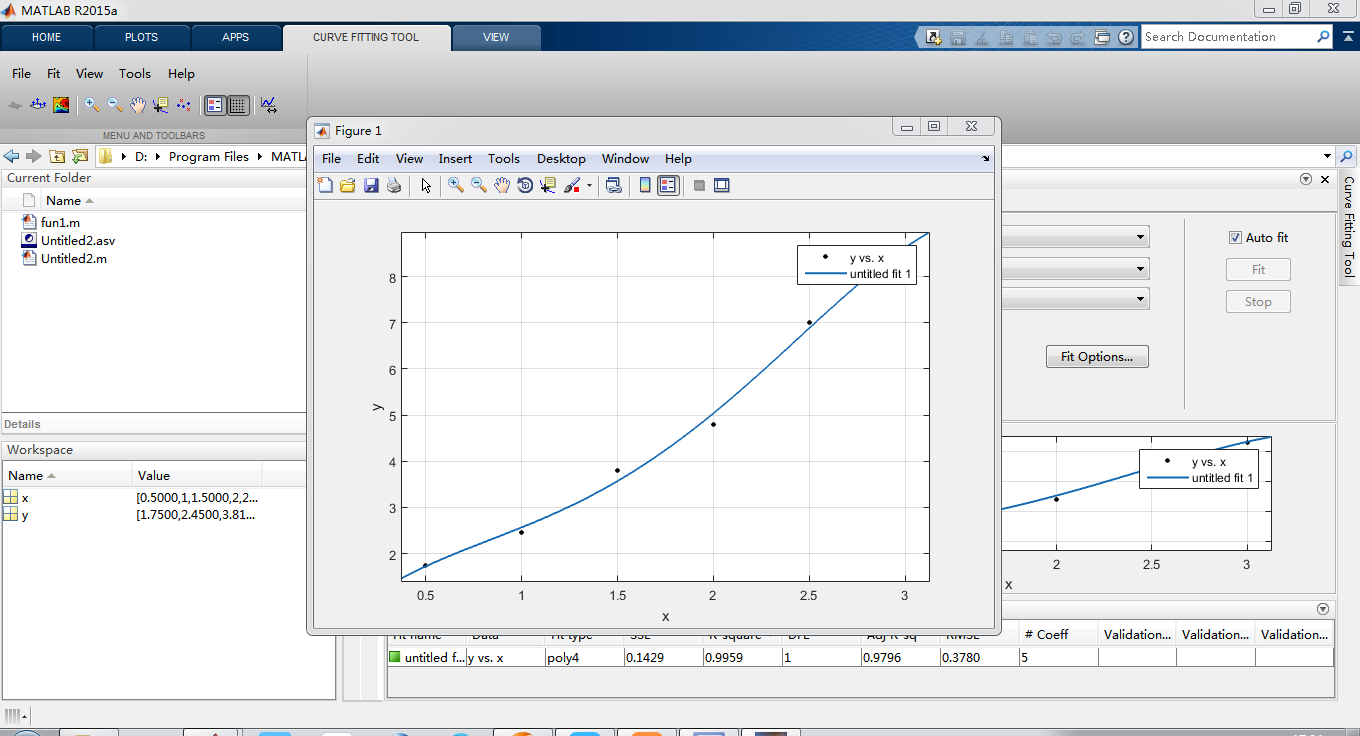12-2709-15
08-1312万+
03-06
07-06
12-162万+
07-284477
03-058986
05-273万+